Part One -- 理解强化学习术语

RL：通过奖励来更新模型参数让模型越来越好。

1、一些名词：

Agent：智能体，做动作的主体

State ：状态

Action

Policy：根据观测到的状态做出决策来控制运动 Π(a|s) = P(A = a | S = s) ，就是给定状态S做出动作A的概率密度。强化学习学的就是policy函数。游戏中：策略随机

State transition：S1 -> action1 -> S2 (获得r1) -> 做action2,得到s1 a1 r1,s2 a2 r2 ... st at rt.

Reward：奖励（定义的好坏非常影响结果）

Return: 由于未来的奖励没有现在的值钱，使用折扣回报(discounted return γ)

$$\vec{U}_{t}=R_{t}+\gamma R_{t+1}+\gamma^{2} R_{t+2}+\gamma^{3} R_{t+3}+...$$

γ为0，1之间，越久远的未来折扣越大权重越低

Ut是随机变量（在t时刻不知道是什么），可以求期望。如抛硬币知道正反有一半概率而不知道下一个会得到什么。

Action Value Function

$$Action\ Value \ Function: \ Q_{\pi}\left(s_{t}, a_{t}\right)=\mathbb{E}\left[U_{t} \mid S_{t}=s_{t}, A_{t}=a_{t}\right]$$

Optimal action-value function

$$Q^{\star}\left(s_{t}, a_{t}\right)=\max _{ \pi} Q_{\pi}\left(s_{t}, a_{t}\right)$$

State-value function:

$$\begin{array}{l} V_{\pi}\left(s_{t}\right)=\mathbb{E}_{A}\left[Q_{\pi}\left(s_{t}, A\right)\right]=\sum_{a} \pi\left(a \mid s_{t}\right) \cdot Q_{\pi}\left(s_{t}, a\right) . \quad \text { (discrete.) }\\ V_{\pi}\left(s_{t}\right)=\mathbb{E}_{A}\left[Q_{\pi}\left(s_{t}, A\right)\right]=\int \pi\left(a \mid s_{t}\right) \cdot Q_{\pi}\left(s_{t}, a\right) d a \cdot \text { (continuous.) } \end{array}$$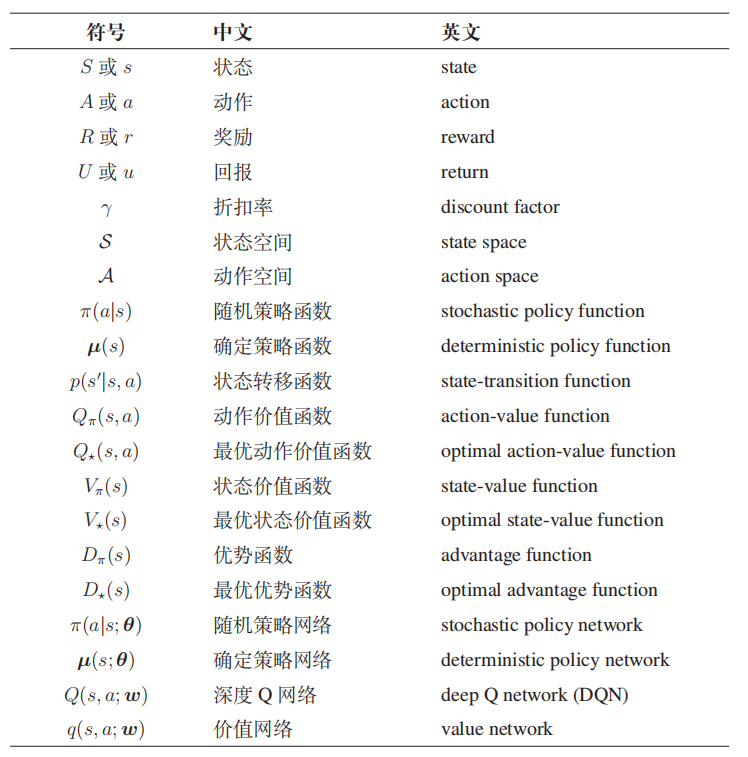2、结合我们：

• Policy-based-learning 学习一个policy函数Π，用policy控制agent做动作，每观测到一个状态st，作为Π函数的输入，会输出每一个动作的概率，按照概率做随机抽样得到at控制agent做动作。
• Value-based-learning，学习的是Optimal action-value ：Q*(s,a)。将当前状态St作为输入，对于每一个动作action都做一个评价，选择Q值最大的来做：

$$a_{t}=\operatorname{argmax}_{a} Q^{\star}\left(s_{t}, a\right)$$

Part Two -- Value-based learning (DQN + TD)

$$Q^{\star}\left(s_{t}, a_{t}\right)=\max _{\pi} \mathbb{E}\left[U_{t} \mid S_{t}=s_{t}, A_{t}=a_{t}\right]$$

1、Deep Q-Network (DQN)

state -Conv卷积层-> feature 特征向量 -Dense全连接层-> 输出打分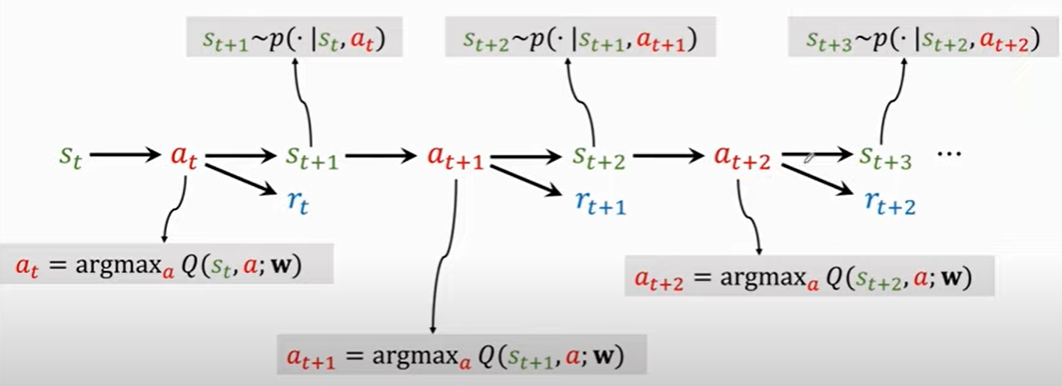2、如何训练DQN -- 用TD算法(Temporal Difference)

1. 当前状态St和当前动作At
2. Qt = Q(s,a;w), Qt 是神经网络的输出，是对每个状态的打分
3. 反向传播求导，PyTorch和TF都可以自动求梯度：

$$\mathbf{d}_{t}=\left.\frac{\partial Q\left(s_{t}, a_{t} ; \mathbf{w}\right)}{\partial \mathbf{w}}\right|_{\mathbf{w}=\mathbf{w}_{t}}$$

1. 环境更新成状态St+1和奖励Rt
2. 求TD target：

TD \ target: \begin{aligned} y_{t} &=r_{t}+\gamma \cdot Q\left(s_{t+1}, a_{t+1} ; \mathbf{w}_{t}\right) \\ &=r_{t}+\gamma \cdot \max _{a} Q\left(s_{t+1}, a ; \mathbf{w}_{t}\right) \end{aligned}

1. 梯度下降更新权重

$$\text { Gradient descent: } \mathbf{w}_{t+1}=\mathbf{w}_{t}-\left.\alpha \cdot \frac{\partial L_{t}}{\partial \mathbf{w}}\right|_{\mathbf{w}=\mathbf{w}_{t}}$$

$$Q\left(s_{t}, a_{t} ; \mathbf{w}\right) \approx r_{t}+\gamma \cdot Q\left(s_{t+1}, a_{t+1} ; \mathbf{w}\right)$$

Part Three-- Policy-based learning (Policy network + Policy gradient)

1、policy network

Π(a|s)：概率密度函数，表示当前状态时某一个action的概率（输入是s)

state -Conv卷积层-> feature 特征向量 -Dense全连接层-> action个数的向量 -> Softmax激活函数 -> 每一个action的概率

$$V\left(s_{t} ; \boldsymbol{\theta}\right)=\sum_{a} \pi\left(a \mid s_{t} ; \boldsymbol{\theta}\right) \cdot Q_{\pi}\left(s_{t}, a\right)$$

$$J(\boldsymbol{\theta})=\mathbb{E}_{S}[V(S ; \boldsymbol{\theta})]$$

• state s

$$Update \ policy \ \boldsymbol{\theta} \leftarrow \boldsymbol{\theta}+\beta \cdot \frac{\partial V(s ; \boldsymbol{\theta})}{\partial \boldsymbol{\theta}}$$

3、Update policy network using policy gradient

1. t时间的状态St
2. 用Π(a|s;θ)随机抽取得到action At
3. 计算价值函数Qt = QΠ(St,At)
• 策略网络控制agent运动，记录agent的轨迹和reward，得到Ut来近似QΠ
• 或者用神经网络来进行函数近似。
1. 求导得到d_θt
2. 近似计算策略梯度
3. 用近似的策略梯度更新策略网络的参数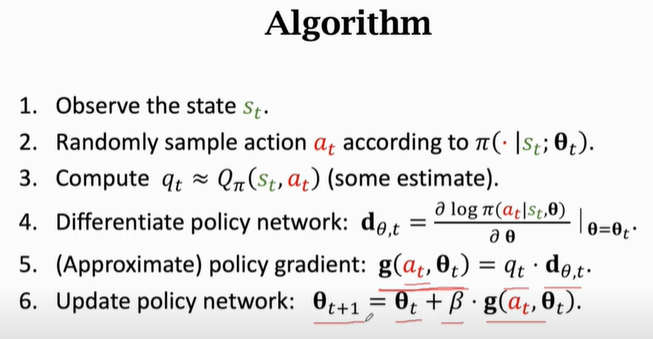Part Four -- Actor-critic method

actor：策略网络，相当于运动员

critic：value network，相当于裁判员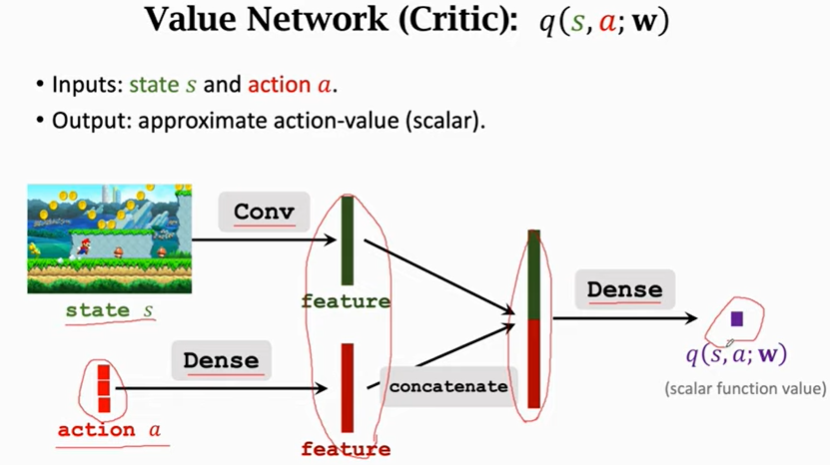Train the networks

• policy network：让运动员得到更高的平均分，更新Π函数，让V函数的值更大。

$$V(s ; \boldsymbol{\theta}, \mathbf{w})=\sum_{a} \pi(a \mid s ; \boldsymbol{\theta}) \cdot q(s, a ; \mathbf{w})$$

• value network：q函数当作裁判，给运动员打分越来越精准。

1. 观察当前状态s
2. 根据策略函数对action进行概率分布，随机取得action
3. 做action更新当前状态St+1得到reward Rt
4. TD 算法更新value network的w。（裁判更准确）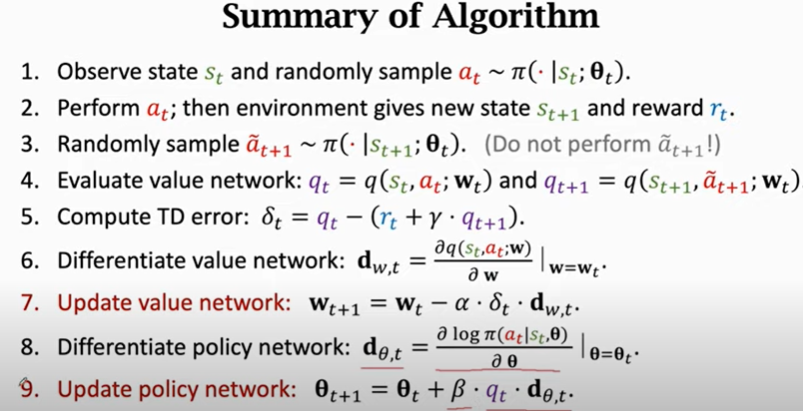Part Five -- AlphaGo

• behavior cloning（模仿学习，非强化学习，监督学习，多分类/回归，将361个状态看成361个类别，计算每种类别的概率，人真实的动作就是标签）